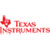# Texas Instruments BAIIPlus PRO Financial Calculator, 10-Digit LCD# Texas Instruments BAIIPlus PRO Financial Calculator, 10-Digit LCD

#### Item #TEXBAIIPLUSPRO

• Features time-saving calculations to make short work of complex equations.
• Ideal for finance, accounting, economics, investment, statistics and other business classes.
• Permitted use on the CFA® exam.
• Rugged brushed metal exterior.\$85.45/EA

Features time-saving calculations to make short work of complex equations. Ideal for finance, accounting, economics, investment, statistics and other business classes. May use on the CFA® exam. Rugged brushed metal exterior.

Power Source(s): Battery; Display Notation: Alphanumeric; Number of Display Digits: 10; Display Characters x Display Lines: 10 x 1.

• Global Product Type Financial Calculators
• Power Source(s) Battery
• Display Notation Alphanumeric
• Number of Display Digits 10
• Display Characters x Display Lines 10 x 1
• Calculators Memory 10K
• Calculators Display Type LCD
• Case Leather-Like Pouch
• Percent Key(s) No
• Fraction Calculations No
• Fraction/Decimal Conversions No
• Decimal Function No
• +/- Switch Key Yes
• Currency Exchange Function No
• Metric Conversion No
• Backspace Key Yes
• Double Zero Key No
• Amortization Yes
• Base Number Calculations No
• Bond Calculations Yes
• Cash Flow Calculations Yes
• Complex Number Calculations No
• Confidence Interval Calculating No
• Cost/Sell/Margin Yes
• Date Calculations Yes
• Depreciation Calculations Yes
• Entry Logic Algebraic (AOS)
• Equation Editor No
• Grand Total Key No
• Hyperbolic Functions No
• Hypothesis Testing No
• Interest Rate Conversion Yes
• Item Count Function No
• Linear Regression Yes
• Loan Calculation Yes
• Logical (Boolean) Operations No
• Markup/Down Key Yes
• Matrices No
• Polar-Rectangular Conversion No
• Probability (Random Number) No
• Simultaneous Equations No
• Square Root Key Yes
• Tax Calculation No
• Time-Value-of-Money Yes
• Time/Date No
• Trig/Log Functions Yes
• Variable Regression Yes
• Variable Statistics One
• Size 3 x 6
• Replacement Batteries Lithium
• Package Includes Battery; Leather-Like Pouch
• Pre-Consumer Recycled Content Percent 0%
• Post-Consumer Recycled Content Percent 0%
• Total Recycled Content Percent 0%
• Country of Origin CN
• Carton Pack Quantity 4
• Pack Quantity 0
• UPC 033317192045
• UNSPSC 44101801